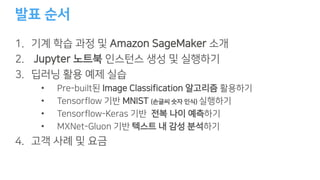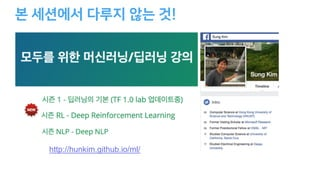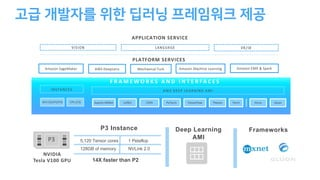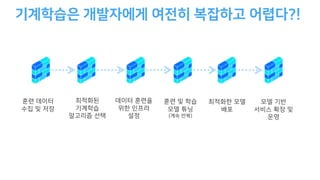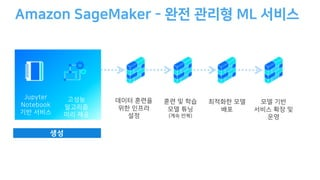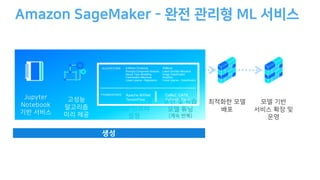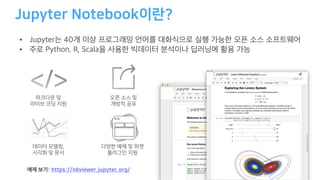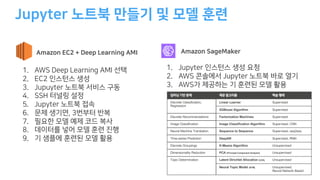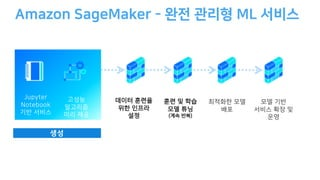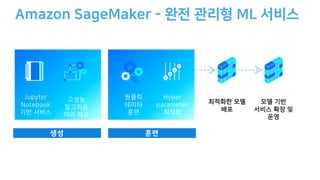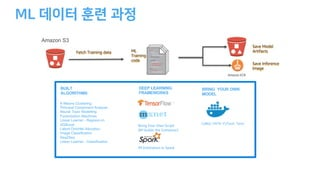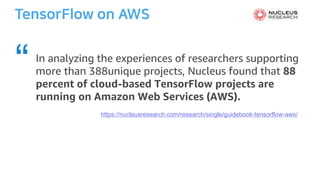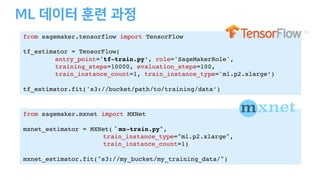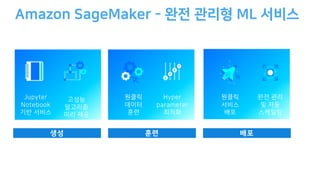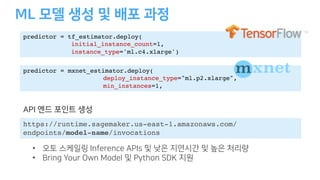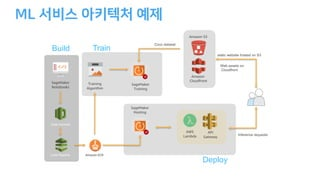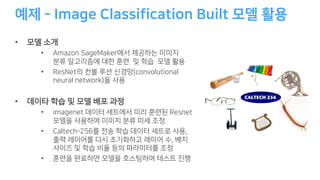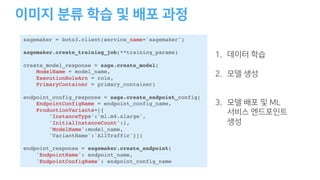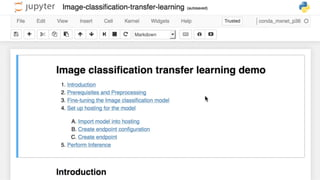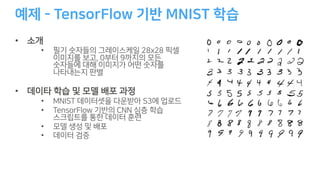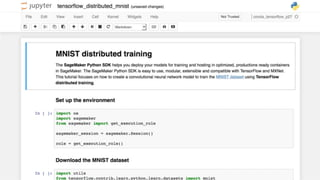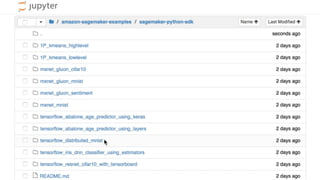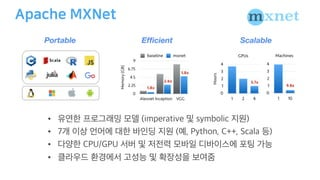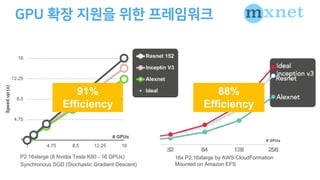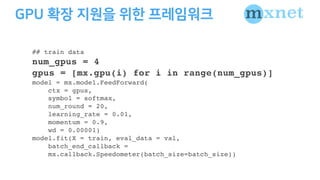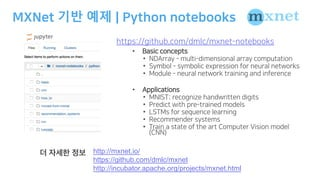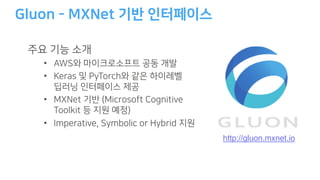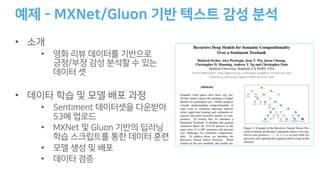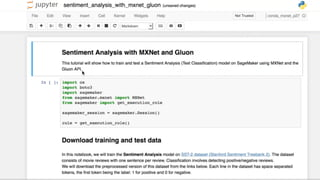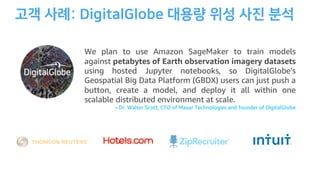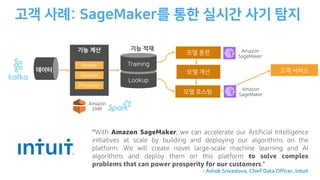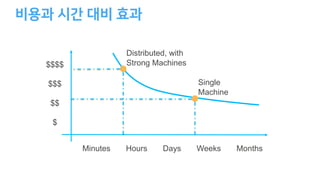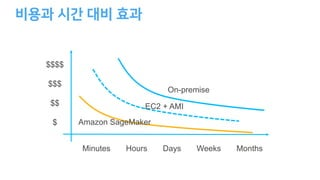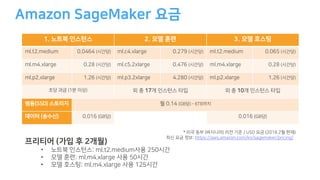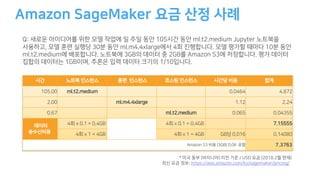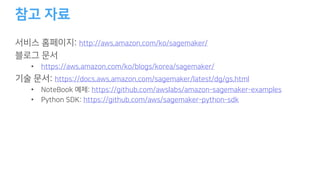1 of 41

### Amazon SageMaker을 통한 손쉬운 Jupyter Notebook 활용하기 - 윤석찬 (AWS 테크에반젤리스트)

2. ( )
3. Nt ) 2 J - AG Tz g g ac X o mu • 1 CC lM o • 3 C 23 e k fr i • 3 C . C s p mw • 4 ygz S Ic b ( MK nP
4. ! http://hunkim.github.io/ml/
5. F R A M E W O R K S A N D I N T E R FA C E S P3 P3 Instance Deep Learning AMI Frameworks PLATFORM SERVICES VISION LANGUAGE VR/IR APPLICATION SERVICE AWS DeepLensAmazon SageMaker Amazon Machine Learning Amazon EMR & SparkMechanical Turk AWS DEEP LEARNING AMI Apache MXNet TensorFlowCaffe2 Torch KerasCNTK PyTorch GluonTheano INSTANCES GPU (G2/P2/P3) CPU (C5) NVIDIA Tesla V100 GPU 5,120 Tensor cores 1 Petaflop 128GB of memory NVLink 2.0 14X faster than P2
6. ! ( )
7. - J N ) ( ((
8. - J N ) ( (( K-Means Clustering Principal Component Analysis Neural Topic Modelling Factorization Machines Linear Learner - Regression XGBoost Latent Dirichlet Allocation Image Classification Seq2Seq Linear Learner - Classification ALGORITHMS Apache MXNet TensorFlow Caffe2, CNTK, PyTorch, Torch FRAMEWORKS
9. / . .. • • , : / / .
10. C A D ,65 .88 387 9 ,41 e t g 2 8 H DM 55 2 8 H u EW L p a L y E n L r 2 8 t g ,65 in 2 8 H S E ,65A C I E L r 2 +2 2 2 J E C Discrete Classification, Regression Linear Learner Supervised XGBoost Algorithm Supervised Discrete Recommendations Factorization Machines Supervised Image Classification Image Classification Algorithm Supervised, CNN Neural Machine Translation Sequence to Sequence Supervised, seq2seq Time-series Prediction DeepAR Supervised, RNN Discrete Groupings K-Means Algorithm Unsupervised Dimensionality Reduction PCA (Principal Component Analysis) Unsupervised Topic Determination Latent Dirichlet Allocation (LDA) Unsupervised Neural Topic Model (NTM) Unsupervised, Neural Network Based
11. - J N ) ( ((
12. - H J N
13. K-Means Clustering Principal Component Analysis Neural Topic Modelling Factorization Machines Linear Learner - Regression XGBoost Latent Dirichlet Allocation Image Classification Seq2Seq Linear Learner - Classification BUILT ALGORITHMS Caffe2, CNTK, PyTorch, Torch IM Estimators in Spark DEEP LEARNING FRAMEWORKS Bring Your Own Script (IM builds the Container) BRING YOUR OWN MODEL ML Training code Fetch Training data Save Model Artifacts Amazon ECR Save Inference Image Amazon S3
14. https://nucleusresearch.com/research/single/guidebook-tensorflow-aws/ In analyzing the experiences of researchers supporting more than 388unique projects, Nucleus found that 88 percent of cloud-based TensorFlow projects are running on Amazon Web Services (AWS). “
15. from sagemaker.tensorflow import TensorFlow tf_estimator = TensorFlow( entry_point='tf-train.py’, role='SageMakerRole', training_steps=10000, evaluation_steps=100, train_instance_count=1, train_instance_type='ml.p2.xlarge’) tf_estimator.fit('s3://bucket/path/to/training/data’) from sagemaker.mxnet import MXNet mxnet_estimator = MXNet(＂mx-train.py", train_instance_type="ml.p2.xlarge", train_instance_count=1) mxnet_estimator.fit("s3://my_bucket/my_training_data/")
16. - H J N
17. - H J N
18. predictor = tf_estimator.deploy( initial_instance_count=1, instance_type='ml.c4.xlarge') predictor = mxnet_estimator.deploy( deploy_instance_type="ml.p2.xlarge", min_instances=1, https://runtime.sagemaker.us-east-1.amazonaws.com/ endpoints/model-name/invocations • BK A ID A • A I
19. SageMaker Notebooks Training Algorithm SageMaker Training Amazon ECR Code Commit Code Pipeline SageMaker Hosting Coco dataset AWS Lambda API Gateway Build Train Deploy static website hosted on S3 Inference requests Amazon S3 Amazon Cloudfront Web assets on Cloudfront
20. - • • (6 - ma wC t k zm h • , s Sc A 5 5 5 r • t h v • 6 t ma , r n t v • ) 5 2 ue h t Ntl i Ntl t h s M v • r oR r g n g
21. sagemaker = boto3.client(service_name='sagemaker') sagemaker.create_training_job(**training_params) create_model_response = sage.create_model( ModelName = model_name, ExecutionRoleArn = role, PrimaryContainer = primary_container) endpoint_config_response = sage.create_endpoint_config( EndpointConfigName = endpoint_config_name, ProductionVariants=[{ 'InstanceType':'ml.m4.xlarge', 'InitialInstanceCount':1, 'ModelName':model_name, 'VariantName':'AllTraffic'}]) endpoint_response = sagemaker.create_endpoint( 'EndpointName': endpoint_name, 'EndpointConfigName': endpoint_config_name 2 . 1 3 .
22. - • • w I n l 9 l NF l l T • C x N S • , C e , • 03 2 x oMs r C • N S • C 8
23. - • S • n aN eb D bF D o • o A b • 13 , K , D • 34 137 4 o T l rC • A •
24. • • • •
25. 1 4.75 8.5 12.25 16 1 4.75 8.5 12.25 16 Speedup(x) # GPUs Resnet 152 Inceptin V3 Alexnet Ideal P2.16xlarge (8 Nvidia Tesla K80 - 16 GPUs) Synchronous SGD (Stochastic Gradient Descent) 91% Efficiency 88% Efficiency 16x P2.16xlarge by AWS CloudFormation Mounted on Amazon EFS # GPUs
26. ## train data num_gpus = 4 gpus = [mx.gpu(i) for i in range(num_gpus)] model = mx.model.FeedForward( ctx = gpus, symbol = softmax, num_round = 20, learning_rate = 0.01, momentum = 0.9, wd = 0.00001) model.fit(X = train, eval_data = val, batch_end_callback = mx.callback.Speedometer(batch_size=batch_size))
27. 기반 예제 B : A I A AA • ( A B • . DD A DD A B A • A A IBD A AD D AD • -A D AD D : D • BB A • -. D A: D : • /D BD D A • - AD C D : • A D • D A D )A B D A A ).. http://mxnet.io/ https://github.com/dmlc/mxnet http://incubator.apache.org/projects/mxnet.html
28. http://gluon.mxnet.io - H • ,X P b fd S • ( C X g NT MI ce • ) A ) A A A K a W • A ,C C a
29. / - • • X M N • e G 3 • N S • / e i • M G •
30. We plan to use Amazon SageMaker to train models against petabytes of Earth observation imagery datasets using hosted Jupyter notebooks, so DigitalGlobe's Geospatial Big Data Platform (GBDX) users can just push a button, create a model, and deploy it all within one scalable distributed environment at scale. - Dr. Walter Scott, CTO of Maxar Technologies and founder of DigitalGlobe
31. EC : A C “With Amazon SageMaker, we can accelerate our Artificial Intelligence initiatives at scale by building and deploying our algorithms on the platform. We will create novel large-scale machine learning and AI algorithms and deploy them on this platform to solve complex problems that can power prosperity for our customers." - Ashok Srivastava, Chief Data Officer, Intuit
32. \$\$\$\$ \$\$\$ \$\$ \$ Minutes Hours Days Weeks Months Single Machine Distributed, with Strong Machines
33. \$\$\$\$ \$\$\$ \$\$ \$ Minutes Hours Days Weeks Months EC2 + AMI Amazon SageMaker On-premise
34. . 2 ) ( 1 G) : - h U 9 8 ).0 h U G) : - h U 8 )/ h U 9 ) 8 .- h U 8 )/ h U D) 8 ( )- h U D* ) 8 )/ h U D) 8 ( )- h U wU ( od k x (. pg g z k x ( pg g z m 553 g n ( 42U -62 o e i (- 42U (- 42U 3A • S a pg g1 G) : cm ) h • 1 8 cm h • g 1 8 cm () h T s u 753 l ) (/ )n r i l t 1 GGD 1 8 8 8 B 9 8 8 D 9 B
35. . 6 3 70 r z d h ( ,w B ) = J 6J =G n h a y * o B B:G = s h ( o B ) = J n *53 )53d 2 : D 8* (53 g c U ( ( w n a w p Q ( , B ) = J /.) ) B B:G = ( () ) ) . B ) = J , *,, tuxp ( 1 53 ( 1 53 . (,,,, ( 1 53 ( 1 53 53 ( ( / 2 : D 8* p *53 / iS m k z e U 84 ) (/ ) x l0 0 : : : D A : = :A=G G D
36. NK : - : / / D • : : - : / : / / B D : . -: : - : / / /: . : • / : - : : : / / / /: • : - : : / / :.